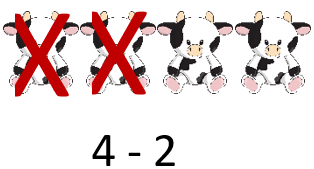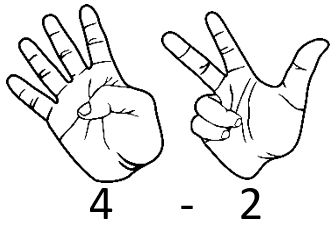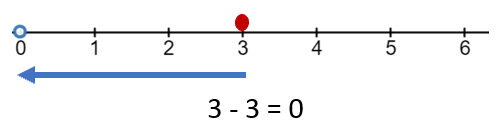# Math Worksheets Land

Math Worksheets For All Ages

# Math Worksheets Land

Math Worksheets For All Ages

Home > Topics >

# Subtraction Worksheets

What is Subtraction? We all know that mastering addition is easier than grasping the concept of subtraction. Children can quickly put two things together to obtain a sum but they struggle with taking away one thing from another. Finding differences between values forms the foundation of many advanced mathematical concepts. Therefore, before they hop on complex concepts, it is necessary that they have a firm grip on finding a basic difference. In mathematics, subtraction is defined as taking away one thing from another. After we process this operation, what remains in the group is less than what was present before the calculation was processed. For example, If you take 3 away for 5 you will be left with 2. To express it mathematically, 6 - 4 =2 A simple subtraction problem usually has three parts. The part from which something is being taken away is known as minuend. The part which is being taken away is known as a subtrahend. The part that you are left with is known as difference. For example, 6 - 4 = 2, the number 6 is the minuend, 4 is the subtrahend and the number 2 is the difference. The worksheets and lessons that you will find here are found mostly mixed in with addition operations in our basic math skills sheets. Those operations are direct opposites of one another and it is always good to make that concept concrete for students.

### Tips For Teaching This Skill

Before we get going on this topic let me just remind you of the parts of subtraction. It will be helpful as you read further. Problems follow the model of:

Minuend - Subtrahend = Difference

We normally approach this basic concept with students in first grade. Collective our staff has taught first grade for over 100 years. So we all got together and discussed the best practices for teaching this skill to students at that age. Here is what we find works:

Always Start with Visuals - This is the immediate point that all of us agreed on. You should always approach this skill as a takeaway method. This is where we give students a problem and model it with objects. We do find that students do better when the numbers of the problem are found below the objects. This probably because it is easier to visually isolate the numbers when they are beneath objects rather than above them. Here is an example of a problem: 4 - 2. We will find a fun object that youngsters will be engaged with. We will use cute toy cows in our example. We teach them that your minuend (4) is the number of baby cows you would start with. Your subtrahend (2) is the number cows that you need to take away. We encourage you to use red slashes to serve as the cross out. We also encourage you to always have them cross out the objects that are most left. We find that this is much easier for them to track long term. So the problem 4-2 would be modelled as:We would then teach them determine the difference by simply counting the number of left-over objects. Some of our teachers prefer starting by using manipulatives and others like visuals such as pictures. Everyone agrees that you will need to use both manipulatives and pictures before you move to anything else. A great manipulative to consider is having students model problems by using their fingers. Remind yourself that you only go as high as 5, but you can put partners together and go to 10. Here is that same problem modelled with fingers.Number Line - Once students have the basic concept through manipulatives and images the natural progression is to use a number line and teach them to count backwards. The minuend is our starting point. We then count backwards the number of times of the subtrahend.This really helps them work on the concept that all this skill is composed of is counting in reverse. Once they have this foundation it is pretty easy to build off. You will then progress on to paper and pencil problems. We also encourage students to either by or make math facts cards and practice 5 to 10 minutes everyday until they are memorized. Even then students will need to still look at them once a week to keep it fresh in their memory.

Unlock all the answers, worksheets, homework, tests and more!
Save Tons of Time! Make My Life Easier Now

## Thanks and Don't Forget To Tell Your Friends!

I would appreciate everyone letting me know if you find any errors. I'm getting a little older these days and my eyes are going. Please contact me, to let me know. I'll fix it ASAP.